ICSE Class 10 Measures of Central Tendency Solution New Pattern

## ICSE Class 10 Measures of Central Tendency Solution By Clarify Knowledge

ICSE Class 10 Measures of Central Tendency Solution New Pattern 2022

## Chapter 24 - Measures of Central Tendency Ex. 24(A)

Question 1

Find the mean of the following set of numbers:

(i) 6, 9, 11, 12 and 7

(ii) 11, 14, 23, 26, 10, 12, 18 and 6Solution 1

(i)

(ii)

Question 2

Marks obtained (in mathematics) by 9 student are given below:

60, 67, 52, 76, 50, 51, 74, 45 and 56

(a) find the arithmetic mean

(b) if marks of each student be increased by 4; what will be the new value of arithmetic mean.Solution 2

(a) Here n = 9

(b)

If marks of each student be increased by 4 then new arithmetic mean will be = 59 + 4 = 63Question 3

Find the mean of the natural numbers from 3 to 12.Solution 3

Numbers between 3 to 12 are 3, 4, 5, 6, 7, 8, 9, 10, 11 and 12.

Here n = 10

Question 4

(a) Find the mean of 7, 11, 6, 5, and 6

(b) If each number given in (a) is diminished by 2, find the new value of mean.Solution 4

(a) The mean of 7, 11, 6, 5 and 6

(b)

If we subtract 2 from each number, then the mean will be 7-2 = 5Question 5

If the mean of 6, 4, 7, 'a' and 10 is 8. Find the value of 'a'Solution 5

No. of terms = 5

Mean = 8

Sum of numbers = 8 x 5 = 40 .(i)

But, sum of numbers = 6+4+7+a+10 = 27+a ..(ii)

From (i) and (ii)

27+a = 40

a = 13Question 6

The mean of the number 6, 'y', 7, 'x' and 14 is 8. Express 'y' in terms of 'x'.Solution 6

No. of terms = 5 and mean = 8

Sum of numbers = 5 x 8 = 40 ..(i)

but sum of numbers = 6+y+7+x+14 = 27+y+x .(ii)

from (i) and (ii)

27 + y + x = 40

x + y = 13

y = 13 - xQuestion 7

The ages of 40 students are given in the following table:

Find the arithmetic mean.Solution 7

Question 8

If 69.5 is the mean of 72, 70, 'x', 62, 50, 71, 90, 64, 58 and 82, find the value of 'x'.Solution 8

No. of terms = 10

Mean = 69.5

Sum of the numbers = 69.5 x 10 = 695 ..........(i)

But sum of numbers = 72+70+x+62+ 50+71+90+64+58+82

= 619 + x ......(ii)

from (i) and (ii)

619 + x = 695

x = 76Question 9

The following table gives the heights of plants in centimeter. If the mean height of plants is 60.95 cm; find the value of 'f'.

Solution 9

Mean = 60.95

Question 10

From the data given below, calculate the mean wage, correct to the nearest rupee.

(i) If the number of workers in each category is doubled, what would be the new mean wage?

(ii) If the wages per day in each category are increased by 60%; what is the new mean wage?

(iii) If the number of workers in each category is doubled and the wages per day per worker are reduced by 40%, what would be the new mean wage?Solution 10

(i) Mean remains the same if the number of workers in each category is doubled.

Mean = 80

(ii) Mean will be increased by 60% if the wages per day per worker is increased by 60%

New mean =(iii) No change in the mean if the number of workers is doubled but if wages per worker is reduced by 40%, then

New mean =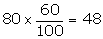Question 11

The contents of 100 match boxes were checked to determine the number of matches they contained.

(i) calculate, correct to one decimal place, the mean number of matches per box.

(ii) Determine how many extra matches would have to be added to the total contents of the 100 boxes to bring the mean up to exactly 39 matches.Solution 11

(i)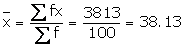(ii) In the second case,

New mean = 39 matches

Total contents = 39 x 100 = 3900

But total number of matches already given = 3813

Number of new matches to be added = 3900 - 3813 = 87Question 12

If the mean of the following distribution is 3, find the value of p.

Solution 12

Question 13

In the following table, ∑f = 200 and mean = 73. Find the missing frequencies f1, and f2.

Solution 13

Question 14

Find the arithmetic mean (correct to the nearest whole-number) by using step-deviation method.

Solution 14

Question 15

Find the mean (correct to one place of decimal) by using short-cut method.

Solution 15

## Chapter 24 - Measures of Central Tendency (Mean, Median, Quartiles and Mode) Exercise Ex. 24(B)

Question 1

The following table gives the ages of 50 students of a class. Find the arithmetic mean of their ages.

Solution 1

Question 2

The following table gives the weekly wages of workers in a factory.

Calculate the mean by using:

(i) Direct Method

(ii) Short - Cut MethodSolution 2

(i) Direct Method

(ii) Short - cut method

Question 3

The following are the marks obtained by 70 boys in a class test:

Calculate the mean by:

(i) Short - cut method

(ii) Step - deviation methodSolution 3

(i) Short - cut method

(ii) Step - deviation method

Here A = 65 and h = 10

Question 4

Find mean by step - deviation method:

Solution 4

Here A = 87.50 and h = 7

Question 5

The mean of the following frequency distribution is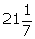. Find the value of 'f'.

Solution 5

Question 6

Using step-deviation method, calculate the mean marks of the following distribution.

Solution 6

Let the assumed mean A= 72.5

Question 7

Using the information given in the adjoining histogram, calculate the mean.

Solution 7

Question 8

If the mean of the following observations is 54, find the value of 'p'.

Solution 8

Here mean = 54 ..(ii)

from (i) and (ii)

Question 9

The mean of the following distribution is 62.8 and the sum of all the frequencies is 50. Find the missing frequencies f1 and f2.

Solution 9

Now,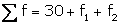andfrom (i)

using (i) and (ii)

Question 10

Calculate the mean of the distribution, given below, using the short cut method :

Solution 10

## Chapter 24 - Measures of Central Tendency (Mean, Median, Quartiles and Mode) Exercise Ex. 24(C)

Question 1

A student got the following marks in 9 questions of a question paper.

3, 5, 7, 3, 8, 0, 1, 4 and 6.

Find the median of these marks.Solution 1

Arranging the given data in descending order:

8, 7, 6, 5, 4, 3, 3, 1, 0

The middle term is 4 which is the 5th term.

Median = 4Question 2

The weights (in kg) of 10 students of a class are given below:

21, 28.5, 20.5, 24, 25.5, 22, 27.5, 28, 21 and 24.

Find the median of their weights.Solution 2

Arranging the given data in descending order:

28.5, 28, 27.5, 25.5, 24, 24, 22, 21, 21, 20.5

The middle terms are 24 and 24, 5th and 6th terms

Question 3

The marks obtained by 19 students of a class are given below:

27, 36, 22, 31, 25, 26, 33, 24, 37, 32, 29, 28, 36, 35, 27, 26, 32, 35 and 28. Find:

(i) median

(ii) lower quartile

(iii) upper quartile

(iv) interquartile rangeSolution 3

Arranging in ascending order:

22, 24, 25, 26, 26, 27, 27, 28, 28, 29, 21, 32, 32, 33, 35, 35, 36, 36, 37

(i) Middle term is 10th term i.e. 29

Median = 29

(ii) Lower quartile =

(iii) Upper quartile =

(iv) Interquartile range = q3 - q1 =35 - 26 = 9Question 4

From the following data, find:

(i) Median

(ii) Upper quartile

(iii) Inter-quartile range

25, 10, 40, 88, 45, 60, 77, 36, 18, 95, 56, 65, 7, 0, 38 and 83Solution 4

Arrange in ascending order:

0, 7, 10, 18, 25, 36, 38, 40, 45, 56, 60, 65, 77, 83, 88, 95

(i) Median is the mean of 8th and 9th term

(ii) Upper quartile =

(iii) Interquartile range =

Question 5

The ages of 37 students in a class are given in the following table:

Find the median.Solution 5

Number of terms = 37

Median =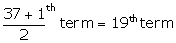Median = 14Question 6

The weight of 60 boys are given in the following distribution table:

Find:

(i) median

(ii) lower quartile

(iii) upper quartile

(iv) interquartile rangeSolution 6

Number of terms = 60

(i) median = the mean of the 30th and the 31st terms

(ii) lower quartile (Q1) =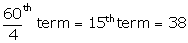(iii) upper quartile (Q3) =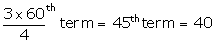(iv) Interquartile range = Q3 - Q= 40 - 38 = 2Question 7

Estimate the median for the given data by drawing an ogive:

Solution 7

Number of terms = 50

Through mark of 25.5 on the y-axis, draw a line parallel to x-axis which meets the curve at A. From A, draw a perpendicular to x-axis, which meets x-axis at B.

The value of B is the median which is 28.Question 8

By drawing an ogive, estimate the median for the following frequency distribution:

Solution 8

Number of terms = 55

Through mark of 28 on the y-axis, draw a line parallel to x-axis which meets the curve at A. From A, draw a perpendicular to x-axis, which meets x-axis at B.

The value of B is the median which is 18.4 kgQuestion 9

From the following cumulative frequency table, find:

(i) median

(ii) lower quartile

(iii) upper quartile

Solution 9

Number of terms = 80

\Median = 40th term.

(i) Median = Through 40th term mark draw a line parallel to the x-axis which meets the curve at A. From A, draw a perpendicular to x-axis which meets it at B.

Value of B is the median = 40

(ii) Lower quartile (Q1) = 20th term = 18

(iii) Upper Quartile (Q3) = 60th term = 66Question 10

In a school, 100 pupils have heights as tabulated below:

Find the median height by drawing an ogive.Solution 10

Number of terms = 100

Through 50th term mark draw a line parallel to the x-axis which meets the curve at A. From A, draw a perpendicular to x-axis which meets it at B.

Value of B is the median = 148Median height = 148cm

## Chapter 24 - Measures of Central Tendency (Mean, Median, Quartiles and Mode) Exercise Ex. 24(D)

Question 1

Find the mode of the following data:

(i) 7, 9, 8, 7, 7, 6, 8, 10, 7 and 6

(ii) 9, 11, 8, 11, 16, 9, 11, 5, 3, 11, 17 and 8Solution 1

(i) Mode = 7

Since 7 occurs 4 times

(ii) Mode = 11

Since it occurs 4 timesQuestion 2

The following table shows the frequency distribution of heights of 50 boys:

Find the mode of heights.Solution 2

Mode is 122 cm because it occur maximum number of times. i.e. frequency is 18.Question 3

Find the mode of following data, using a histogram:

Solution 3

Mode is in 20-30, because in this class there are 20 frequencies.Question 4

The following table shows the expenditure of 60 boys on books. Find the mode of their expenditure:

Solution 4

Mode is in 30-35 because it has the maximum frequency.Question 5

Find the median and mode for the set of numbers:

2, 2, 3, 5, 5, 5, 6, 8 and 9Solution 5which is 5.

Mode = 5 because it occurs maximum number of times.Question 6

A boy scored following marks in various class tests during a term; each test being marked out of 20.

15, 17, 16, 7, 10, 12, 14, 16, 19, 12 and 16

(i) What are his modal marks?

(ii) What are his median marks?

(iii) What are his total marks?

(iv) What are his mean marks?Solution 6

Arranging the given data in ascending order:

7, 10, 12, 12, 14, 15, 16, 16, 16, 17, 19

(i) Mode = 16 as it occurs maximum number of times.

(ii)(iii)Total marks = 7+10+12+12+14+15+16+16+16+17+19 =

154

(iv)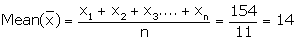Question 7

Find the mean, median and mode of the following marks obtained by 16 students in a class test marked out of 10 marks.

0, 0, 2, 2, 3, 3, 3, 4, 5, 5, 5, 5, 6, 6, 7 and 8.Solution 7

(i)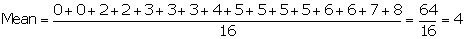(ii) Median = mean of 8th and 9th term

(iii) Mode = 5 as it occurs maximum number of times.Question 8

At a shooting competition the score of a competitor were as given below:

(i) What was his modal score?

(ii) What was his median score?

(iii) What was his total score?

(iv) What was his mean score?Solution 8

(i) Modal score = 4 as it has maximum frequency 7.

(ii)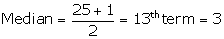(iii) Total score = 80

(iv)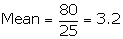## Chapter 24 - Measures of Central Tendency (Mean, Median, Quartiles and Mode) Exercise Ex. 24(E)

Question 1

The following distribution represents the height of 160 students of a school.

Draw an ogive for the given distribution taking 2 cm = 5 cm of height on one axis and 2 cm = 20 students on the other axis. Using the graph, determine:

1. The median height.
2. The interquartile range.
3. The number of students whose height is above 172 cm.

Solution 1

Taking Height of student along x-axis and cumulative frequency along y-axis we will draw an ogive.

(i)

Through mark for 80, draw a parallel line to x-axis which meets the curve; then from the curve draw a vertical line which meets the x-axis at the mark of 157.5.

(ii)Since, number of terms = 160

(iii)Through mark for 172 on x-axis, draw a vertical line which meets the curve; then from the curve draw a horizontal line which meets the y-axis at the mark of 145.

The number of students whose height is above 172 cm

= 160 - 144 = 16Question 2

Draw ogive for the data given below and from the graph determine: (i) the median marks.

(ii) the number of students who obtained more than 75% marks.

Solution 2

Scale:

1cm = 10 marks on X axis

1cm = 20 students on Y axis

(i)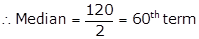Through mark 60, draw a parallel line to x-axis which meets the curve at A. From A, draw a perpendicular to x-axis meeting it at B.

The value of point B is the median = 43

(ii) Total marks = 100

75% of total marks =marks

The number of students getting more than 75% marks = 120 - 111 = 9 students.Question 3The mean of 1, 7, 5, 3, 4 and 4 is m. The numbers 3, 2, 4, 2, 3, 3 and p have mean m-1 and median q. Find p and q.Solution 3

Mean of 1, 7, 5, 3, 4 and 4 =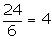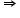m=4

Now, mean of 3, 2, 4, 2, 3, 3 and p = m-1 = 4-1 = 3

Therefore, 17+p = 3 x n …. Where n = 7

17+p = 21

p = 4

Arranging in ascending order:

2, 2, 3, 3, 3, 3, 4, 4

Mean = 4th term = 3

Therefore, q = 3Question 4

In a malaria epidemic, the number of cases diagnosed were as follows:

On what days do the mode and upper and lower quartiles occur?Solution 4

(i) Mode = 5th July as it has maximum frequencies.

(ii) Total number of terms = 206

Upper quartile =Lower quartile =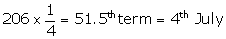Question 5

The income of the parents of 100 students in a class in a certain university are tabulated below.

(i) Draw a cumulative frequency curve to estimate the median income.

(ii) If 15% of the students are given freeships on the basis of the basis of the income of their parents, find the annual income of parents, below which the freeships will be awarded.

(iii) Calculate the Arithmetic mean.Solution 5

We plot the points (8, 8), (16, 43), (24, 78), (32, 92) and (40, 100) to get the curve as follows:

At y = 50, affix A.

Through A, draw a horizontal line meeting the curve at B.

Through B, a vertical line is drawn which meets OX at M.

OM = 17.6 units

Hence, median income = 17.6 thousands

Question 6

The marks of 20 students in a test were as follows:

2, 6, 8, 9, 10, 11, 11, 12, 13, 13, 14, 14, 15, 15, 15, 16, 16, 18, 19 and 20.

Calculate:

(i) the mean (ii) the median (iii) the modeSolution 6

Arranging the terms in ascending order:

2, 6, 8, 9, 10, 11, 11, 12, 13, 13, 14, 14, 15, 15, 15, 16, 16, 18, 19, 20

Number of terms = 20

(i)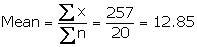(ii)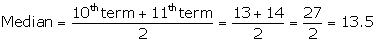(iii) Mode = 15 as it has maximum frequencies i.e. 3Question 7

The marks obtained by 120 students in a mathematics test is given below:

Draw an ogive for the given distribution on a graph sheet. Use a suitable scale for your ogive. Use your ogive to estimate:

(i)the median

(ii)the number of students who obtained more than 75% in test.

(iii)the number of students who did not pass in the test if the pass percentage was 40.

(iv)the lower quartileSolution 7

(i)Through mark 60.5, draw a parallel line to x-axis which meets the curve at A, From A draw a perpendicular to x-axis meeting it at B.

The value of point B is the median = 43

(ii) Number of students who obtained up to 75% marks in the test = 110

Number of students who obtained more than 75% marks in the test = 120 - 110 = 10

(iii) Number of students who obtained less than 40% marks in the test = 52 (from the graph; x=40, y=52)

(iv) Lower quartile = Q1 =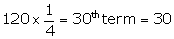Question 8

Using a graph paper, draw an ogive for the following distribution which shows a record of the width in kilograms of 200 students.

Use your ogive to estimate the following:

(i) The percentage of students weighing 55 kg or more

(ii) The weight above which the heaviest 30% of the student fall

(iii) The number of students who are

(a) underweight

(b) overweight,

if 55.70 kg is considered as standard weight.Solution 8

(i) Number of students weighing more than 55 kg = 200-44 = 156

Therefore, percentage of students weighing 55 kg or more

(ii) 30% of students =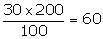Heaviest 60students in weight = 9 + 21 + 30 = 60

weight = 65 kg ( from table)

(iii) (a) underweight students when 55.70 kg is standard = 46 (approx) from graph

(b) overweight students when 55.70 kg is standard = 200- 55.70 = 154 (approx) from graphQuestion 9

The distribution, given below, shows the marks obtained by 25 students in an aptitude test. Find the mean, median and mode of the distribution.

Solution 9

Number of terms = 25

(i) Mean =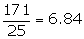(ii)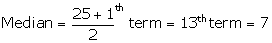(iii) Mode = 6 as it has maximum frequencies i.e. 6Question 10

The mean of the following distribution is 52 and the frequency of class interval 30-40 is 'f'. Find f.

Solution 10

Question 11

The monthly income of a group of 320 employees in a company is given below:

Draw an ogive of the given distribution on a graph paper taking 2 cm = Rs 1000 on one axis and 2 cm = 50 employees on the other axis. From the graph determine :

(i) the median wage.

(ii) number of employees whose income is below Rs 8500.

(iii) if salary of a senior employee is above Rs 11,500, find the number of senior employees in the company.

(iv) the upper quartile.Solution 11

Number of employees = 320

(i)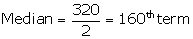Through mark 160, draw a parallel line to x-axis which meets the curve at A, From A draw a perpendicular to x-axis meeting it at B.

The value of point B is the median = Rs 9.3 thousands

(ii) The number of employees with income below Rs 8500 = 95 (approx from the graph)

(iii) Number of employees with income below Rs 11500 = 305 (approx from the graph)

Therefore number of employees (senior employees) = 320-305 =15

(iv) Upper quartile =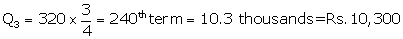Question 12

A mathematics aptitude test of 50 students was recorded as follows:

Draw a histogram for the above data using a graph paper and locate the mode.Solution 12

(i)Draw the histogram

(ii) In the highest rectangle which represents modal class draw two lines AC and BD intersecting at P.

(iii) From P, draw a perpendicular to x-axis meeting at Q.

(iv) Value of Q is the mode = 82 (approx)Question 13

Marks obtained by 200 students in an examination are given below:

Draw an ogive of the given distribution on a graph paper taking 2 cm = 10 marks on one axis and 2 cm = 20 students on the other axis. Using the graph:

(i) the median marks.

(ii) number of students who failed if minimum marks required to pass is 40

(iii) if scoring 85 and more marks is considered as grade one, find the number of students who secured grade one in the examination.Solution 13

Number of students = 200

(i)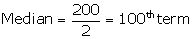Through mark 100, draw a parallel line to x-axis which meets the curve at A, From A draw a perpendicular to x-axis meeting it at B.

The value of point B is the median = 57 marks (approx)

(ii) The number of students who failed (if minimum marks required to pass is 40)= 46 (approx from the graph)

(iii) The number of students who secured grade one in the examination = 200 - 188 = 12 (approx from the graph)Question 14

The marks obtained by 40 students in a short assessment is given below, where a and b are two missing data.

If mean of the distribution is 7.2, find a and b.Solution 14

Question 15

Find the mode and the median of the following frequency distribution.

Solution 15

Since the frequency for x = 14 is maximum.

So Mode = 14.

According to the table it can be observed that the value of x from the 13th term to the 17th term is 13.

So the median = 13.Question 16

The median of the observations 11, 12, 14, (x - 2) (x + 4), (x + 9), 32, 38, 47 arranged in ascending order is 24. Find the value of x and hence find the mean.Solution 16

Question 17

The number 6, 8, 10, 12, 13 and x are arranged in an ascending order. If the mean of the observations is equal to the median, find the value of x.Solution 17

Question 18

(Use a graph paper for this question). The daily pocket expenses of 200 students in a school are given below :

Draw a histogram representing the above distribution and estimate the mode from the graph.Solution 18

Histogram is as follows:

In the highest rectangle which represents modal class draw two lines AC and BD intersecting at E.

From E, draw a perpendicular to x-axis meeting at L.

Value of L is the mode. Hence, mode = 21.5Question 19

The marks obtained by 100 students in a mathematics test are given below :

Draw an ogive for the given distribution on a graph sheet.

Use a scale of 2 cm = 10 units on both the axes.

Use the ogive to estimate :

(i) Median

(ii) Lower quartile

(iii) Number of students who obtained more than 85% marks in the test.

(iv) Number of students failed, if the pass percentage was 35.Solution 19

The ogive is as follows:

Question 20

The mean of following numbers is 68. Find the value of 'x'.

45, 52, 60, x, 69, 70, 26, 81 and 94.

Hence, estimate the median.Solution 20

Question 21

The marks of 10 students of a class in an examination arranged in ascending order is as follows:

13, 35, 43, 46, x, x + 4, 55, 61, 71, 80

If the median marks is 48, find the value of x. Hence, find the mode of the given data.Solution 21

Here the number of observations i. e is 10, which is even.'

So, the given data is 13, 35, 43, 46, 46, 50, 55, 61, 71, 80.

In the given data, 46 occurs most frequently.

∴ Mode = 46 Question 22

The daily wages of 80 workers in a project are given below.

Use a graph paper to draw an ogive for the above distribution. (Use a scale of 2 cm = Rs. 50 on x - axis and 2 cm = 10 workers on y - axis). Use your ogive to estimate.

i. the median wages of the workers.

ii. the lower quartile wage of workers.

iii. the number of workers who earn more than Rs. 625 daily.Solution 22

The cumulative frequency table of the given distribution is as follows:

The ogive is as follows:

Number of workers = n = 80

1) Median =term = 40th term, draw a horizontal line which meets the curve at point A.

Draw vertical line parallel to y axis from A to meet x axis at B.

The value of point B is 605.

2) Lower quartile (Q1)=term=20th term = 550

3) Through mark of point 625 on x axis draw a vertical line which meets the graph at point C Then through point C, draw a horizontal line which meets the y axis at the mark of 50.

Thus, the number of workers that earn more than Rs. 625 daily = 80 - 50 = 30Question 23

The histogram below represents the scores obtained by 25 students in a Mathematics mental test. Use the data to:

i. Frame a frequency distribution table.

ii. To calculate mean.

iii. To determine the Modal class.

Solution 23

i. The frequency distribution table is as follows:

ii.

iii. Here the maximum frequency is 8 which is corresponding to class 20 - 30.

Hence, the modal class is 20 - 30.

error: Content is protected !!